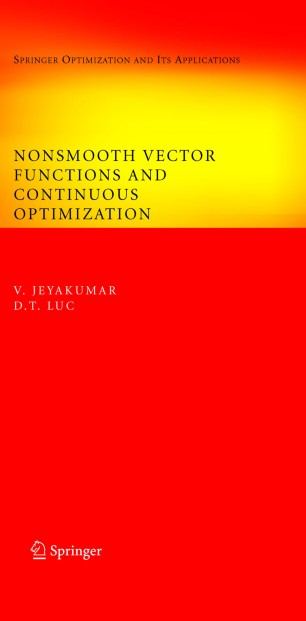# Nonsmooth Vector Functions and Continuous Optimization

• V. Jeyakumar
• D.T. LUCBook

Part of the Optimization and Its Applications book series (SOIA, volume 10)

1. Front Matter
Pages I-X
2. V. Jeyakumar, D.T. LUC
Pages 1-56
3. V. Jeyakumar, D.T. LUC
Pages 57-97
4. V. Jeyakumar, D.T. LUC
Pages 99-142
5. V. Jeyakumar, D.T. LUC
Pages 143-206
6. V. Jeyakumar, D.T. LUC
Pages 207-254
7. Back Matter
Pages 255-269

### Introduction

A recent significant innovation in mathematical sciences has been the progressive use of nonsmooth calculus, an extension of the differential calculus, as a key tool of modern analysis in many areas of mathematics, operations research, and engineering. Focusing on the study of nonsmooth vector functions, this book presents a comprehensive account of the calculus of generalized Jacobian matrices and their applications to continuous nonsmooth optimization problems and variational inequalities in finite dimensions.

The treatment is motivated by a desire to expose an elementary approach to nonsmooth calculus by using a set of matrices to replace the nonexistent Jacobian matrix of a continuous vector function. Such a set of matrices forms a new generalized Jacobian, called pseudo-Jacobian. A direct extension of the classical derivative that follows simple rules of calculus, the pseudo-Jacobian provides an axiomatic approach to nonsmooth calculus, a flexible tool for handling nonsmooth continuous optimization problems.

Illustrated by numerous examples of known generalized derivatives, the work may serve as a valuable reference for graduate students, researchers, and applied mathematicians who wish to use nonsmooth techniques and continuous optimization to model and solve problems in mathematical programming, operations research, and engineering. Readers require only a modest background in undergraduate mathematical analysis to follow the material with minimal effort.

### Keywords

Derivative Minimum Operations Research Operator SOIA calculus differential equation mathematical programming model optimization programming

#### Authors and affiliations

• V. Jeyakumar
• 1
• D.T. LUC
• 2
1. 1.University of New South WalesSydneyAustralia
2. 2.University of AvignonAvignonFrance